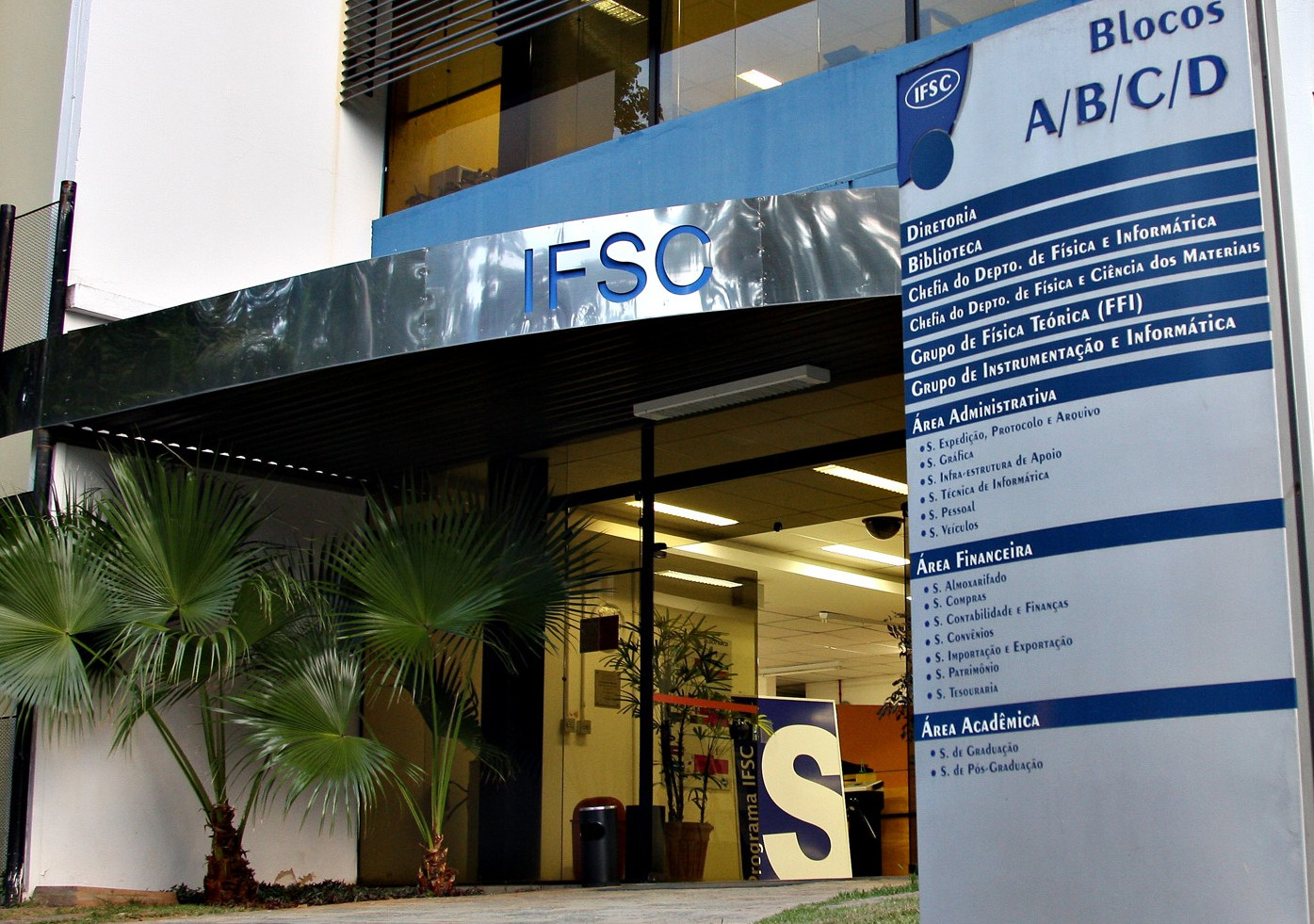# Abstract

The correlation functions of certain random antiferromagnetic spin-1/2 critical chains.
GETELINA, João Carlos de Andrade; HOYOS, José Abel.Abstract: We study the spin-spin correlations in two distinct random critical XX spin-1/2 chain models via exact diagonalization. For the well-known case of uncorrelated random coupling constants, we study the non-universal numerical prefactors and relate them to the corresponding Lyapunov exponent of the underlying single-parameter scaling theory. We have also obtained the functional form of the correct scaling variables important for describing even the strongest finite-size effects. Finally, with respect to the distribution of the correlations, we have numerically determined that they converge to a universal (disorder-independent) non-trivial and narrow distribution when properly rescaled by the spin-spin separation distance in units of the Lyapunov exponent. With respect to the less known case of correlated coupling constants, we have determined the corresponding exponents and shown that both typical and mean correlations functions decay algebraically with the distance. While the exponents of the transverse typical and mean correlations are nearly equal, implying a narrow distribution of transverse correlations, the longitudinal typical and mean correlations critical exponents are quite distinct implying much broader distributions. Further comparisons between these models are given.
 European Physical Journal B v. 93, p. 2-1-2-13 - Ano: 2020 Fator de Impacto: 1,440@article={002984286,author = {GETELINA, João Carlos de Andrade; HOYOS, José Abel.},title={The correlation functions of certain random antiferromagnetic spin-1/2 critical chains},journal={European Physical Journal B},note={v. 93, p. 2-1-2-13},year={2020}}